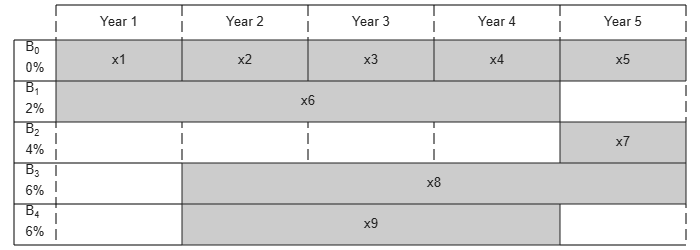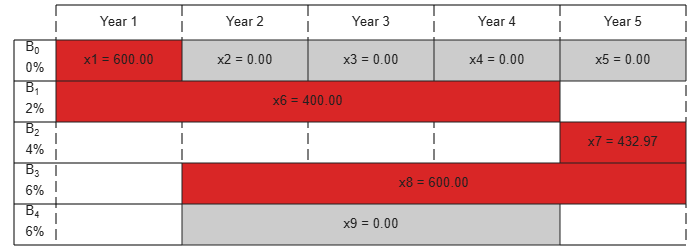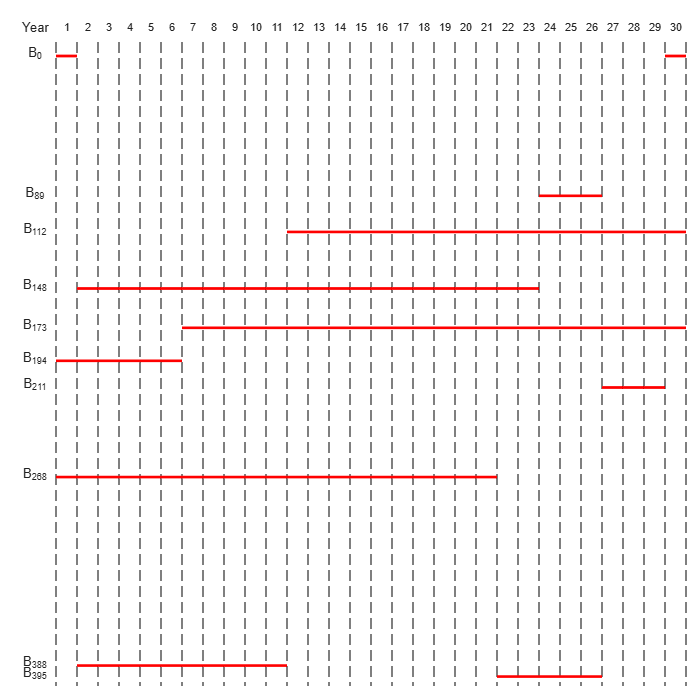# Maximize Long-Term Investments Using Linear Programming: Problem-Based

This example shows how to use the problem-based approach to solve an investment problem with deterministic returns over a fixed number of years `T`. The problem is to allocate your money over available investments to maximize your final wealth. For the solver-based approach, see Maximize Long-Term Investments Using Linear Programming: Solver-Based.

### Problem Formulation

Suppose that you have an initial amount of money `Capital_0` to invest over a time period of `T` years in `N` zero-coupon bonds. Each bond pays a fixed interest rate, compounds the investment each year, and pays the principal plus compounded interest at the end of a maturity period. The objective is to maximize the total amount of money returned after `T` years.

You can include a constraint that no single investment is more than a certain fraction of your total capital at the time of the investment.

This example shows the problem setup on a small case first, and then formulates the general case.

You can model this as a linear programming problem. Therefore, to optimize your wealth, formulate the problem using the optimization problem approach.

### Introductory Example

• The starting amount to invest `Capital_0` is \$1000.

• The time period `T` is 5 years.

• The number of bonds `N` is 4.

• To model uninvested money, have one option B0 available every year that has a maturity period of 1 year and a interest rate of 0%.

• Bond 1, denoted by B1, can be purchased in year 1, has a maturity period of 4 years, and interest rate of 2%.

• Bond 2, denoted by B2, can be purchased in year 5, has a maturity period of 1 year, and interest rate of 4%.

• Bond 3, denoted by B3, can be purchased in year 2, has a maturity period of 4 years, and interest rate of 6%.

• Bond 4, denoted by B4, can be purchased in year 2, has a maturity period of 3 years, and interest rate of 6%.

By splitting up the first option B0 into 5 bonds with maturity period of 1 year and interest rate of 0%, this problem can be equivalently modeled as having a total of 9 available bonds, such that for `k=1..9`

• Entry `k` of vector `PurchaseYears` represents the beginning of the year that bond `k` is available for purchase.

• Entry `k` of vector `Maturity` represents the maturity period ${m}_{k}$ of bond `k`.

• Entry `k` of vector `MaturityYears` represents the end of the year that bond `k` is available for sale.

• Entry `k` of vector `InterestRates` represents the percentage interest rate ${\rho }_{k}$ of bond `k`.

Visualize this problem by horizontal bars that represent the available purchase times and durations for each bond.

```% Time period in years T = 5; % Number of bonds N = 4; % Initial amount of money Capital_0 = 1000; % Total number of buying oportunities nPtotal = N+T; % Purchase times PurchaseYears = [1;2;3;4;5;1;5;2;2]; % Bond durations Maturity = [1;1;1;1;1;4;1;4;3]; % Bond sale times MaturityYears = PurchaseYears + Maturity - 1; % Interest rates in percent InterestRates = [0;0;0;0;0;2;4;6;6]; % Return after one year of interest rt = 1 + InterestRates/100; plotInvestments(N,PurchaseYears,Maturity,InterestRates)```### Decision Variables

Represent your decision variables by a vector `x`, where `x(k)` is the dollar amount of investment in bond `k`, for `k = 1,...,9`. Upon maturity, the payout for investment `x(k)` is

`$x\left(k\right)\left(1+{\rho }_{k}/100{\right)}^{{m}_{k}}.$`

Define ${\beta }_{k}=1+{\rho }_{k}/100$ and define ${r}_{k}$ as the total return of bond `k`:

`${r}_{k}=\left(1+{\rho }_{k}/100{\right)}^{{m}_{k}}={\beta }_{k}^{{m}_{k}}.$`

```x = optimvar('x',nPtotal,'LowerBound',0); % Total returns r = rt.^Maturity;```

### Objective Function

The goal is to choose investments to maximize the amount of money collected at the end of year `T`. From the plot, you see that investments are collected at various intermediate years and reinvested. At the end of year `T`, the money returned from investments 5, 7, and 8 can be collected and represents your final wealth:

`$\underset{x}{\mathrm{max}}{x}_{5}{r}_{5}+{x}_{7}{r}_{7}+{x}_{8}{r}_{8}$`

Create an optimization problem for maximization, and include the objective function.

```interestprob = optimproblem('ObjectiveSense','maximize'); interestprob.Objective = x(5)*r(5) + x(7)*r(7) + x(8)*r(8);```

### Linear Constraints: Invest No More Than You Have

Every year, you have a certain amount of money available to purchase bonds. Starting with year 1, you can invest the initial capital in the purchase options ${x}_{1}$ and ${x}_{6}$, so:

`${x}_{1}+{x}_{6}={Capital}_{0}$`

Then for the following years, you collect the returns from maturing bonds, and reinvest them in new available bonds to obtain the system of equations:

`$\begin{array}{ll}{x}_{2}+{x}_{8}+{x}_{9}& ={r}_{1}{x}_{1}\\ {x}_{3}& ={r}_{2}{x}_{2}\\ {x}_{4}& ={r}_{3}{x}_{3}\\ {x}_{5}+{x}_{7}& ={r}_{4}{x}_{4}+{r}_{6}{x}_{6}+{r}_{9}{x}_{9}\end{array}$`

```investconstr = optimconstr(T,1); investconstr(1) = x(1) + x(6) == Capital_0; investconstr(2) = x(2) + x(8) + x(9) == r(1)*x(1); investconstr(3) = x(3) == r(2)*x(2); investconstr(4) = x(4) == r(3)*x(3); investconstr(5) = x(5) + x(7) == r(4)*x(4) + r(6)*x(6) + r(9)*x(9); interestprob.Constraints.investconstr = investconstr;```

### Bound Constraints: No Borrowing

Because each amount invested must be positive, each entry in the solution vector $x$ must be positive. Include this constraint by setting a lower bound on the solution vector $x$. There is no explicit upper bound on the solution vector.

`x.LowerBound = 0;`

### Solve the Problem

Solve this problem with no constraints on the amount you can invest in a bond. The interior-point algorithm can be used to solve this type of linear programming problem.

```options = optimoptions('linprog','Algorithm','interior-point'); [sol,fval,exitflag] = solve(interestprob,'options',options)```
```Solving problem using linprog. Solution found during presolve. ```
```sol = struct with fields: x: [9×1 double] ```
```fval = 1.2625e+03 ```
```exitflag = OptimalSolution ```

### Visualize the Solution

The exit flag indicates that the solver found an optimal solution. The value `fval`, returned as the second output argument, corresponds to the final wealth. Look at the final sum of investments, and the investment allocation over time.

```fprintf('After %d years, the return for the initial \$%g is \$%g \n',... T,Capital_0,fval);```
```After 5 years, the return for the initial \$1000 is \$1262.48 ```
`plotInvestments(N,PurchaseYears,Maturity,InterestRates,sol.x)`### Optimal Investment with Limited Holdings

To diversify your investments, you can choose to limit the amount invested in any one bond to a certain percentage `Pmax` of the total capital that year (including the returns for bonds that are currently in their maturity period). You obtain the following system of inequalities:

`$\begin{array}{ll}{x}_{1}& \le Pmax×{Capital}_{0}\\ {x}_{2}& \le Pmax×\left({\beta }_{1}{x}_{1}+{\beta }_{6}{x}_{6}\right)\\ {x}_{3}& \le Pmax×\left({\beta }_{2}{x}_{2}+{\beta }_{6}^{2}{x}_{6}+{\beta }_{8}{x}_{8}+{\beta }_{9}{x}_{9}\right)\\ {x}_{4}& \le Pmax×\left({\beta }_{3}{x}_{3}+{\beta }_{6}^{3}{x}_{6}+{\beta }_{8}^{2}{x}_{8}+{\beta }_{9}^{2}{x}_{9}\right)\\ {x}_{5}& \le Pmax×\left({\beta }_{4}{x}_{4}+{\beta }_{6}^{4}{x}_{4}+{\beta }_{8}^{3}{x}_{8}+{\beta }_{9}^{3}{x}_{9}\right)\\ {x}_{6}& \le Pmax×{Capital}_{0}\\ {x}_{7}& \le Pmax×\left({\beta }_{4}{x}_{4}+{\beta }_{6}^{4}{x}_{4}+{\beta }_{8}^{3}{x}_{8}+{\beta }_{9}^{3}{x}_{9}\right)\\ {x}_{8}& \le Pmax×\left({\beta }_{1}{x}_{1}+{\beta }_{6}{x}_{6}\right)\\ {x}_{9}& \le Pmax×\left({\beta }_{1}{x}_{1}+{\beta }_{6}{x}_{6}\right)\end{array}$`

```% Maximum percentage to invest in any bond Pmax = 0.6; constrlimit = optimconstr(nPtotal,1); constrlimit(1) = x(1) <= Pmax*Capital_0; constrlimit(2) = x(2) <= Pmax*(rt(1)*x(1) + rt(6)*x(6)); constrlimit(3) = x(3) <= Pmax*(rt(2)*x(2) + rt(6)^2*x(6) + rt(8)*x(8) + rt(9)*x(9)); constrlimit(4) = x(4) <= Pmax*(rt(3)*x(3) + rt(6)^3*x(6) + rt(8)^2*x(8) + rt(9)^2*x(9)); constrlimit(5) = x(5) <= Pmax*(rt(4)*x(4) + rt(6)^4*x(6) + rt(8)^3*x(8) + rt(9)^3*x(9)); constrlimit(6) = x(6) <= Pmax*Capital_0; constrlimit(7) = x(7) <= Pmax*(rt(4)*x(4) + rt(6)^4*x(6) + rt(8)^3*x(8) + rt(9)^3*x(9)); constrlimit(8) = x(8) <= Pmax*(rt(1)*x(1) + rt(6)*x(6)); constrlimit(9) = x(9) <= Pmax*(rt(1)*x(1) + rt(6)*x(6)); interestprob.Constraints.constrlimit = constrlimit;```

Solve the problem by investing no more than 60% in any one asset. Plot the resulting purchases. Notice that your final wealth is less than the investment without this constraint.

`[sol,fval] = solve(interestprob,'options',options);`
```Solving problem using linprog. Minimum found that satisfies the constraints. Optimization completed because the objective function is non-decreasing in feasible directions, to within the function tolerance, and constraints are satisfied to within the constraint tolerance. ```
```fprintf('After %d years, the return for the initial \$%g is \$%g \n',... T,Capital_0,fval);```
```After 5 years, the return for the initial \$1000 is \$1207.78 ```
`plotInvestments(N,PurchaseYears,Maturity,InterestRates,sol.x)`### Model of Arbitrary Size

Create a model for a general version of the problem. Illustrate it using `T` = 30 years and 400 randomly generated bonds with interest rates from 1 to 6%. This setup results in a linear programming problem with 430 decision variables.

```% For reproducibility rng default % Initial amount of money Capital_0 = 1000; % Time period in years T = 30; % Number of bonds N = 400; % Total number of buying oportunities nPtotal = N + T; % Generate random maturity durations Maturity = randi([1 T-1],nPtotal,1); % Bond 1 has a maturity period of 1 year Maturity(1:T) = 1; % Generate random yearly interest rate for each bond InterestRates = randi(6,nPtotal,1); % Bond 1 has an interest rate of 0 (not invested) InterestRates(1:T) = 0; % Return after one year of interest rt = 1 + InterestRates/100; % Compute the return at the end of the maturity period for each bond: r = rt.^Maturity; % Generate random purchase years for each option PurchaseYears = zeros(nPtotal,1); % Bond 1 is available for purchase every year PurchaseYears(1:T)=1:T; for i=1:N % Generate a random year for the bond to mature before the end of % the T year period PurchaseYears(i+T) = randi([1 T-Maturity(i+T)+1]); end % Compute the years where each bond reaches maturity at the end of the year MaturityYears = PurchaseYears + Maturity - 1;```

Compute the times when bonds can be bought or sold. The `buyindex` matrix holds the potential purchase times, and the `sellindex` matrix holds the potential sales times for each bond.

```buyindex = false(nPtotal,T); % allocate nPtotal-by-T matrix for ii = 1:T buyindex(:,ii) = PurchaseYears == ii; end sellindex = false(nPtotal,T); for ii = 1:T sellindex(:,ii) = MaturityYears == ii; end```

Set up the optimization variables corresponding to the bonds.

`x = optimvar('x',nPtotal,1,'LowerBound',0);`

Create the optimization problem and objective function.

```interestprob = optimproblem('ObjectiveSense','maximize'); interestprob.Objective = sum(x(sellindex(:,T)).*r(sellindex(:,T)));```

For convenience, create a temporary array xBuy, whose columns represent the bonds we can buy at each time period.

`xBuy = repmat(x,1,T).*double(buyindex);`

Similarly, create a temporary array xSell, whose columns represent the bonds we can sell at each time period.

`xSell = repmat(x,1,T).*double(sellindex);`

The return generated for selling these bounds is

`xReturnFromSell = xSell.*repmat(r,1,T);`

Create the constraint that the amount you invest in each time period is the amount that you sold in the previous time period.

```interestprob.Constraints.InitialInvest = sum(xBuy(:,1)) == Capital_0; interestprob.Constraints.InvestConstraint = sum(xBuy(:,2:T),1) == sum(xReturnFromSell(:,1:T-1),1);```

### Solution with No Holding Limit

Solve the problem.

```tic [sol,fval,exitflag] = solve(interestprob,'options',options);```
```Solving problem using linprog. Minimum found that satisfies the constraints. Optimization completed because the objective function is non-decreasing in feasible directions, to within the function tolerance, and constraints are satisfied to within the constraint tolerance. ```
`toc`
```Elapsed time is 0.033693 seconds. ```

How well did the investments do?

```fprintf('After %d years, the return for the initial \$%g is \$%g \n',... T,Capital_0,fval);```
```After 30 years, the return for the initial \$1000 is \$5167.58 ```

### Solution with Limited Holdings

To create constraints that limit the fraction of investments in each asset, set up a matrix that keeps track of the active bonds at each time. To express the constraint that each investment must be less than `Pmax` times the total value, set up a matrix that keeps track of the value of each investment at each time. For this larger problem, set the maximum fraction that can be held to 0.4.

`Pmax = 0.4;`

Create an `active` matrix corresponding to times when a bond can be held, and a `cactive` matrix that holds the cumulative duration of each active bond. So the value of bond `j` at time `t` is `x(j)*(rt^cactive)`.

```active = double(buyindex | sellindex); for ii = 1:T active(:,ii) = double((ii >= PurchaseYears) & (ii <= MaturityYears)); end cactive = cumsum(active,2); cactive = cactive.*active;```

Create the matrix whose entry (j,p) represents the value of bond j at time period p:

`bondValue = repmat(x, 1, T).*active.*(rt.^(cactive));`

Determine the total value of the investments at each time interval so you can impose the constraint on limited holdings. `mvalue` is the money invested in all the bonds at the end of each time period, an `nPtotal`-by-`T` matrix.moneyavailable is the sum over the bonds of the money invested at the beginning of the time period, meaning the value of the portfolio at each time.

```constrlimit = optimconstr(nPtotal,T); constrlimit(:,1) = xBuy(:,1) <= Pmax*Capital_0; constrlimit(:,2:T) = xBuy(:,2:T) <= repmat(Pmax*sum(bondValue(:,1:T-1),1), nPtotal, 1).*double(buyindex(:,2:T)); interestprob.Constraints.constrlimit = constrlimit;```

Solve the problem with limited holdings.

```tic [sol,fval,exitflag] = solve(interestprob,'options',options);```
```Solving problem using linprog. Minimum found that satisfies the constraints. Optimization completed because the objective function is non-decreasing in feasible directions, to within the function tolerance, and constraints are satisfied to within the constraint tolerance. ```
`toc`
```Elapsed time is 0.627319 seconds. ```
```fprintf('After %d years, the return for the initial \$%g is \$%g \n',... T,Capital_0,fval);```
```After 30 years, the return for the initial \$1000 is \$5095.26 ```

To speed up the solver, try the dual-simplex algorithm.

```options = optimoptions('linprog','Algorithm','dual-simplex'); tic [sol,fval,exitflag] = solve(interestprob,'options',options);```
```Solving problem using linprog. Optimal solution found. ```
`toc`
```Elapsed time is 0.149014 seconds. ```
```fprintf('After %d years, the return for the initial \$%g is \$%g \n',... T,Capital_0,fval);```
```After 30 years, the return for the initial \$1000 is \$5095.26 ```

In this case, the dual-simplex algorithm took less time to obtain the same solution.

### Qualitative Result Analysis

To get a feel for the solution, compare it to the amount `fmax` that you would get if you could invest all of your starting money in one bond with a 6% interest rate (the maximum interest rate) over the full 30 year period. You can also compute the equivalent interest rate corresponding to your final wealth.

```% Maximum amount fmax = Capital_0*(1+6/100)^T; % Ratio (in percent) rat = fval/fmax*100; % Equivalent interest rate (in percent) rsol = ((fval/Capital_0)^(1/T)-1)*100; fprintf(['The amount collected is %g%% of the maximum amount \$%g '... 'that you would obtain from investing in one bond.\n'... 'Your final wealth corresponds to a %g%% interest rate over the %d year '... 'period.\n'], rat, fmax, rsol, T)```
```The amount collected is 88.7137% of the maximum amount \$5743.49 that you would obtain from investing in one bond. Your final wealth corresponds to a 5.57771% interest rate over the 30 year period. ```
`plotInvestments(N,PurchaseYears,Maturity,InterestRates,sol.x,false)`